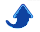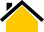# Dr. Phillip M. Feldman

Python is a high-level, general-purpose, open-source, interpreted programming language whose development has been a collaborative effort involving a large community. Although sometimes described as a scripting language, Python is also well-suited for large-scale applications. Its built-in support for numerical/scientific computing is rather weak, but this was rectified by the addition of the NumPy and SciPy packages. In the following, when I refer to Python, I'm implicitly including such widely-used Python packages as NumPy, SciPy, and matplotlib.

"NumPy [initially released in 2005] is an extension to the Python programming language, adding support for large, multi-dimensional arrays and matrices, along with a large library of high-level mathematical functions to operate on these arrays. ... Because Python is currently implemented as an interpreter, mathematical algorithms written in it often run slower than compiled equivalents. NumPy seeks to address this problem for numerical algorithms by providing multidimensional arrays and functions and operators that operate efficiently on arrays. Thus any algorithm that can be expressed primarily as operations on arrays and matrices can run almost as quickly as the equivalent C code." [from http://en.wikipedia.org/wiki/NumPy]

Python and Matlab both use dynamic typing and are higher-level than programming languages such as C/C++ and Java, in the sense that the manipulation of objects such as matrices and arrays requires far less code in Python and Matlab. Python's support for numerical/scientific computing is roughly comparable to that of Matlab, and there is in fact really no third contender in this category. (Mathematica is an excellent tool for symbolic math, but is severely deficient as a programming language).

## News and Miscellany

4 Oct., 2015: I've recently started digging into the IPython Interactive Computing and Visualization Cookbook by Cyrille Rossant. (The snippets of sample content at http://ipython-books.github.io/cookbook are hard to understand without the context). Although I'm in the main opposed to computer programming cookbooks, this is far better than most books in that genre. The author begins each chapter with a brief introduction that combines background and motivation. The examples are well designed and explained.

Last update: 16 May, 2016